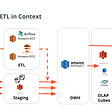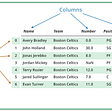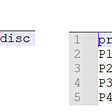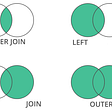# Leaning pandas. Data manipulation and visualization. (COVID-19 in Scotland — statistics)

`import pandas as pd data = pd.read_excel ('https://www.nrscotland.gov.uk/files//statistics/covid19/weekly-deaths-by-location-age-sex.xlsx', sheet_name='Data', skiprows=4, skipfooter=2, usecols='A:F', header=None, names=['week','location','sex','age','cause','deaths'] ) data`
`select sum(deaths) from data group by week`
`import numpy as np data.groupby('week').agg({'deaths': np.sum})`
`data.groupby('week').agg({'deaths': np.sum}).plot()`
`data.groupby('week').agg({'deaths': np.sum}).plot(figsize=(24,10),title='Total deaths by week')`
`data_1519 = pd.read_excel ('https://www.nrscotland.gov.uk/files//statistics/covid19/weekly-deaths-by-location-age-group-sex-15-19.xlsx', sheet_name='Data', skiprows=4, skipfooter=2, usecols='A:F', header=None, names=['year','week','location','sex','age','deaths']) data_1519`
`data_1519['cause'] = 'Pre-COVID-19'`
`neworder =['year','week','location','sex','age','cause','deaths'] data_1519 = data_1519.reindex(columns=neworder) data_1519`
`data_2021 = data # let's keep original DataFrame as is and work with a copy from now on data_2021['year'] = data_2021.week.str.slice(0,2).astype(int) data_2021['week'] = data_2021.week.str.slice(3,5).astype(int) data_2021`
`neworder =['year','week','location','sex','age','cause','deaths'] data_2021 = data_2021.reindex(columns=neworder) data_2021`
`data_2021.groupby(['year','week']).agg({'deaths': np.sum}).plot(title='Total deaths by week')`
`data_1519.groupby(['year','week']).agg({'deaths': np.sum}).plot(title='Total deaths by week')`
`totals_1519 = data_1519.groupby(['year','week']).agg({'deaths': np.sum}) totals_1519['deaths_15'] = None totals_1519['deaths_16'] = None totals_1519['deaths_17'] = None totals_1519['deaths_18'] = None totals_1519['deaths_19'] = None totals_1519`
`totals_1519.shape (261,6)`
`totals_1519.reset_index(inplace=True) totals_1519`
`totals_1519.loc[totals_1519['year']==15,'deaths_15']=totals_1519['deaths'] totals_1519.loc[totals_1519['year']==16,'deaths_16']=totals_1519['deaths'] totals_1519.loc[totals_1519['year']==17,'deaths_17']=totals_1519['deaths'] totals_1519.loc[totals_1519['year']==18,'deaths_18']=totals_1519['deaths'] totals_1519.loc[totals_1519['year']==19,'deaths_19']=totals_1519['deaths'] totals_1519`
`totals_1519.plot(x='week',y=['deaths_15','deaths_16','deaths_17','deaths_18','deaths_19'],title='Total deaths by week')`
`totals_2021 = data_2021.groupby(['year','week']).agg({'deaths': np.sum})  totals_2021['deaths_20'] = None totals_2021['deaths_21'] = None totals_2021.reset_index(inplace=True) totals_2021.loc[totals_2021['year']==20,'deaths_20']=totals_2021['deaths'] totals_2021.loc[totals_2021['year']==21,'deaths_21']=totals_2021['deaths'] totals_2021`
`totals = totals_1519 totals=totals.append(totals_2021,ignore_index=True) totals`
`totals.plot(x='week',y=['deaths_15','deaths_16','deaths_17','deaths_18','deaths_19','deaths_20','deaths_21'],title='Total deaths by week',figsize=(24,10))`
`totals[totals['week']<=9].plot(x='week',y=['deaths_15','deaths_16','deaths_17','deaths_18','deaths_19','deaths_20','deaths_21'],title='Total deaths in Scotland by week',figsize=(24,10))`

--

--

--

## More from Valentine Kulikov

Love podcasts or audiobooks? Learn on the go with our new app.

## Backpressure## Ask the Operatives (Issue #3)## 9 Free API Development Tools to Build REST APIs | TechAffinity## 5 Compelling Social Media Scraping Tools Available On The Web## 10 Podcasts For Web Developers That Will Elevate Your Skills## More from Medium## Pandas Tutorial Part III — Modifying Data within the DataFrame## Deriving useful metrics from Pandas data frame comparison## Joining dataframe in pandas